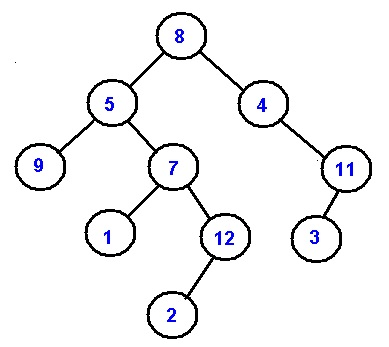Q:

# Root to leaf Path Sum

Given a Binary Tree T and a sum S, write a program to check whether there is a root to leaf path in that tree with the input sum S.

Example:Fig: Binary Tree

In the above example, the root is 8 & the leaf nodes are 9,1,2,3. There are many root to leaf paths like:
8->5->9
8->5->7->1
8->5->7->12->2
And so on.

The sum for a root to leaf path is the sum of all intermediate nodes, the root & leaf node, i.e., sum of all the nodes on the path. Like for the first path in the above example the root to leaf path sum is 22 (8+5+9)

Our program must be to determine whether the given sum is same as anythe root to leaf path sum. There may be case that more than one root to leaf path has exactly same sum of S, but that’s not of any concern, Our function is a Boolean function to return only yes or not on basis of input.

Let for an example input:

S= 26 & T be the above binary tree in example

Our program should return "YES" as there is a root to leaf path exists which is 8->4->11->3

S=8 & T be the above binary tree in example

Our program should return "NO" as there is no root to leaf path exists which has sum 8

Algorithm

We need to construct a Boolean function hasRootToLeafSum () which will accept a sum value S & a binary tree T

We can implement the above function using recursion. The idea is to subtract current node value & continue to check for both subtrees recursively until we reach the base case. The base case can be of two type:

1. Root node for the subtrees are NULL & sum is 0 too. This is a valid terminating step. We return TRUE here.
2. The sum is 0 & we have reached a leaf node. This is of course the valid terminating step. We return TRUE here.

FunctionhasRootToLeafSum (sum value S, root of Binary tree T)

```1.  Set path to FALSE. Path represent the Boolean
variable which determines whether there is a root to leaf pathor not.
2.  Consider the base cases.
•   If (root==NULL&&S==0)  //case a
return true;
•   Subtract the root node value & check whether we have reached
the leaf node with remaining S, 0.
If (S==0 && root->left==NULL &&root->right==NULL)
return true;

3.	Recursively check for both right & left subtrees.
If(root->left) //for left subtree
path=path||hasRootToLeafSum (root->left, S);

If (root->right)//for right subtree
path=path||hasRootToLeafSum (root->right, S);

4.	Return path ( FALSE or TRUE)

If the function return FALSE then no path is found else there is a path.```

## C++ implementation of Root to leaf Path Sum

``````#include<bits/stdc++.h>
using namespace std;

class tree{ //tree node
public:
int data;
tree *left;
tree *right;
};

bool hasRootToLeafSum(tree *root, int s){
bool path=false; //declare boolean variable path
//base condition checking
if(root==NULL && s==0)
return true;

s-=root->data; //subtract current root value

//checking whether leaf node reached and remaining sum =0
if(s==0 && root->left==NULL && root->right==NULL)
return true;
//recursively done for both subtrees
if(root->left){//for left subtree
path=path||hasRootToLeafSum(root->left, s);
}
if(root->right){//for right subtree
path=path||hasRootToLeafSum(root->right, s);
}
return path;
}

tree* newnode(int data){  //creating new nodes
tree* node = (tree*)malloc(sizeof(tree));
node->data = data;
node->left = NULL;
node->right = NULL;
return(node);
}

int main() {
//**same tree is builted as shown in example**
cout<<"tree in the example is build here"<<endl;
//building the tree like as in the example
tree *root=newnode(8);
root->left= newnode(5);
root->right= newnode(4);
root->right->right=newnode(11);
root->right->right->left=newnode(3);
root->left->left=newnode(9);
root->left->right=newnode(7);
root->left->right->left=newnode(1);
root->left->right->right=newnode(12);
root->left->right->right->left=newnode(2);

int s;

cout<<"enter input sum S......"<<endl;
cin>>s;

if(hasRootToLeafSum(root,s))//if there exists such a path
cout<<"A root to leaf path with this sum  exists"<<endl;
else
cout<<"No such a path exists"<<endl;

return 0;
}
``````

Output

```First run:
tree in the example is build here
enter input sum S......
26
A root to leaf path with this sum  exists

Second run:
tree in the example is build here
enter input sum S......
8
No such a path exists```